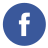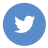### eval in javascript

javascript

eval() is a built-in JavaScript function that allows you to execute a string of JavaScript code as if it were part of the current scope. It takes a string as an argument, which is the JavaScript code to be executed.

Here’s an example of how to use eval():

``````var x = 2;
var y = 3;
var result = eval('x + y'); // result = 5``````

In this example, we define two variables x and y, and then use eval() to execute a string of JavaScript code that adds x and y together. The result of the evaluation is stored in the result variable.

However, eval() should be used with caution because it can be a security risk. If you pass user-generated code to eval(), it can execute malicious code and expose your application to vulnerabilities. For this reason, it is generally recommended to avoid using eval() and instead find alternative solutions to execute dynamic code.

If you need to execute code dynamically, consider using Function(), which allows you to create a function from a string of JavaScript code. The Function() constructor creates a new function object from a string containing the function’s body. Here’s an example of how to use Function():

``````var x = 2;
var y = 3;
var addFunction = new Function('a', 'b', 'return a + b');
var result = addFunction(x, y); // result = 5``````

In this example, we use the Function() constructor to create a new function that takes two arguments and returns their sum. We then call the function with the x and y variables as arguments to get the result. This approach is safer than using eval() because the code is parsed as a function rather than as a string of executable code.